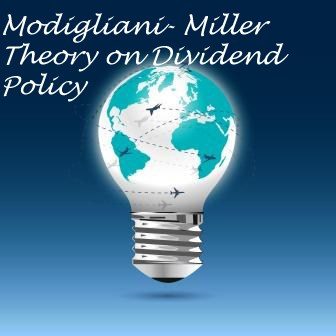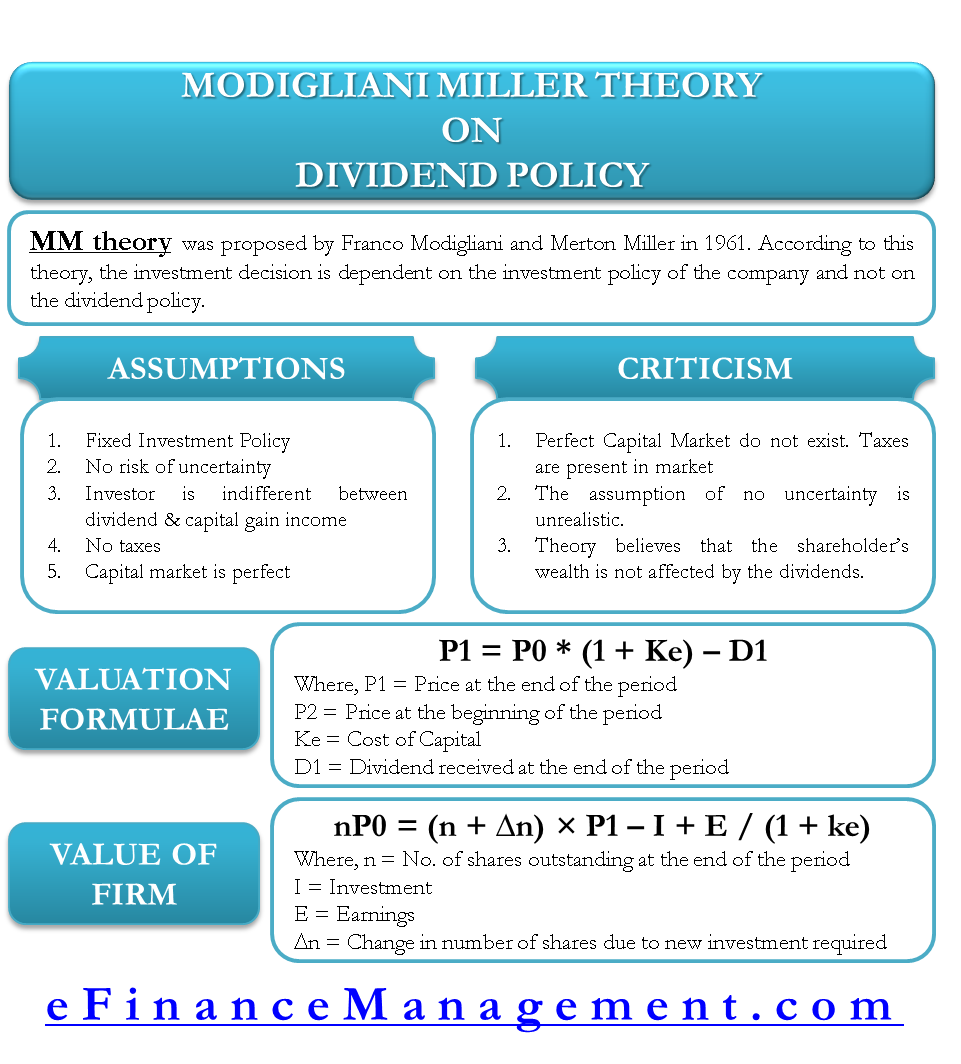data-full-width-responsive="true">

# Modigliani- Miller Theory on Dividend Policy

Modigliani – Miller theory is a major proponent of ‘Dividend Irrelevance’ notion. According to this concept, investors do not pay any importance to the dividend history of a company and thus, dividends are irrelevant in calculating the valuation of a company. This theory is in direct contrast to the ‘Dividend Relevance’ theory which deems dividends to be important in the valuation of a company.

## Crux of Modigliani-Miller Model

Modigliani – Miller theory was proposed by Franco Modigliani and Merton Miller in 1961. They were the pioneers in suggesting that dividends and capital gains are equivalent when an investor considers returns on investment. The only thing that impacts the valuation of a company is its earnings, which is a direct result of the company’s investment policy and the future prospects. So, according to this theory, once the investment policy is known to the investor, he will not need any additional input on the dividend history of the company. The investment decision is, thus, dependent on the investment policy of the company and not on the dividend policy.

Modigliani – Miller theory goes a step further and illustrates the practical situations where dividends are not relevant to investors. Irrespective of whether a company pays a dividend or not, the investors are capable enough to make their own cash flows from the stocks depending on theirneed for the cash. If the investor needs more money than the dividend he received, he can always sell a part of his investments to make up for the difference. Likewise, if an investor has no present cash requirement, he can always reinvest the received dividend in the stock. Thus, the Modigliani – Miller theory firmly states that the dividend policy of a company has no influence on the investment decisions of the investors.

This theory also believes that dividends are irrelevant by the arbitrage argument. By this logic, the dividends distribution to shareholders is offset by the external financing. Due to the distribution of dividends, the price of the stock decreases and will nullify the gain made by the investors because of the dividends.

## Assumptions of the Model

Modigliani – Miller theory is based on the following assumptions:

### Perfect Capital Markets

This theory believes in the existence of ‘perfect capital markets’. It assumes that all the investors are rational, they have access to free information, there are no flotation or transaction costs and no large investor to influence the market price of the share.

### No Taxes

There is no existence of taxes. Alternatively, both dividends and capital gains are taxed at the same rate.

### Fixed Investment Policy

The company does not change its existing investment policy. It means whatever may be the dividend payment, the company will make investment as it has already decided upon. If the company is going to pay more amount of dividend, then it will more equity shares and vice versa.

### No Risk of Uncertainty

All the investors are certain about the future market prices and the dividends. This means that the same discount rate is applicable for all types of stocks in all time periods.

### Investor is indifferent between dividend income and capital gain income

It is assumed that investor is indifferent between dividend income and capital gain income. It means if he requires total return of Rs. 500, he may get Rs. 200 dividend income and Rs. 300 as capital gain income or reverse, in either of the case he gets equal satisfaction.

## Valuation Formula and its Denotations

Modigliani – Miller’s valuation model is based on the assumption of same discount rate/rate of return applicable to all the stocks.

P1 = P0 * (1 + ke) – D1

Where,

P1 = market price of the share at the end of a period

P0 = market price of the share at the beginning of a period

ke = cost of capital

D1 = dividends received at the end of a period## Explanation of Modigliani – Miller’s model

Modigliani – Miller’s model can be used to calculate the market price of the share at the end of a period, if the share price at the beginning of the period, dividends and the cost of capital are known.

Share price at the beginning of the year is Rs. 150. The discount rate applicable to the company is 10%. The company declares Rs. 10 as dividends at the end of a year. Market price of the share at the end of one year using the Modigliani – Miller’s model can be found as under.

Here, P0 = 150

ke = 10%

D1 = 10

Market price of the stock = P1 = 150 * (1 + .10) – 10 = 150 *1.1 – 10 = 155.

## As per M M Approach value of entire firm / company can be found as under:-

nP0 = (n + ∆ n) × P1 – I + E / (1 + ke)

Explanation of formula:-

1. Retained Earning = E – n D1

E = Earning

n = Number of Outstanding Equity shares at the beginning of the year

D1= Dividend Paid to existing shareholders at the end of year

1. New Issue of Equity Share Capital (Rs.) = I – Retained earning

= I – {E – n D1}

= I – E + nD1

I = Investment to be made at the end of the year

1. New Issue of Equity shares at the end of the year (∆n)

New Issue of Equity Shares at the end of year = ∆n × P1

So, we can say that

∆n × P1 = New Issue of Equity Share Capital (Rs.)

∆n × P1 = I – E + nD1

Now, explanation of formula starts,

As we know that for one equity share :-

P1 = P0 * (1 + ke) – D1

P0 = D1 + P1 / (1 + ke)

Now, in above equation, n is multiplied on both sides, so instead of one share, it will become value of firm:-

nP0 = nD1 + nP1 / (1 + ke)

In order to derive a formula, ∆n × P1 is added and subtracted to right hand side equation:-

nP0 = nD1 + nP1 + ∆n × P1 –  ∆n × P1/ (1 + ke)

Now, P1 is taken common from nP1 and ∆n  P1

nP0 = nD1 + (n + ∆n) × P1 –  ∆n × P1/ (1 + ke)

∆n × P1 is replaced with  I – E + nD1

nP0 = nD1 + (n + ∆n) × P1 –  {I – E + nD1}/ (1 + ke)

Now, we open bracket of I – E + nD1

nP0 = nD1 + (n + ∆n) × P1 –  I + E – nD1/ (1 + ke)

nD1 is cancelled from both sides;

So, now we are left with following formula :-

nP0 = + (n + ∆n) × P1 –  I + E / (1 + ke)

## Criticism of Modigliani Miller’s Model

Modigliani – Miller theory on dividend policy suffers from the following limitations:

• Perfect capital markets do not exist. Taxes are present in the capital markets.
• According to this theory, there is no difference between internal and external financing. However, if the flotation costs of new issues are considered, it is false.
• This theory believes that the shareholder’s wealth is not affected by the dividends. However, there are transaction costs associated with the selling of shares to make cash inflows. This makes the investors prefer dividends.
• The assumption of no uncertainty is unrealistic. The dividends are relevant under the certain conditions as well.Summary

Modigliani – Miller theory of dividend policy is an interesting and a different approach to the valuation of shares. It is a popular model which believes in the irrelevance of the dividends. However, the policy suffers from various important limitations and thus, is critiqued regarding its assumptions.

References:

Articles:

Last updated on : February 8th, 2019

** Disclaimer: This post may contain Affiliate Links marked as ** and we may earn a commission on sale.

1.gurpreet mann
2.Neelan
3.Xia